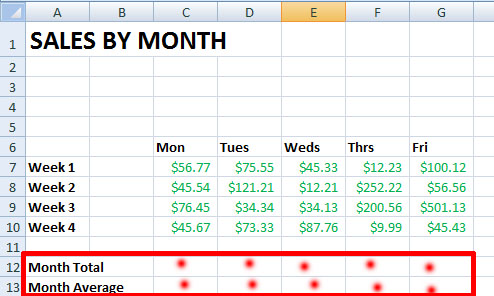Home Microsoft Office Microsoft Excel Using Autosum with Microsoft Excel

# Using Autosum with Microsoft Excel

0
1284

#### Many people use Microsoft Excel to crunch numbers and keep track of sales. It’s ability to insert and utilizing formulas is incredible. This tutorial and screencast demonstrates Excel’s powerful autosum feature to calculate the sum and the average of a column of numbers.

Autosum is a feature that will pick cells to add for you and write its own formula. In this tutorial I will show you how to use autosum to add numbers and find the average of a list of numbers.

1. Create your spreadsheet and label it respectively with columns and cells open for your calculations to appear in. I have a spot for Total Earned (Month Total) and Total Average (Month Average) for each day of the week. The sums will go in all the cells with red dots.2. To find the Month Total for Monday click once on cell C12.3. Select the Home tab on the Excel Ribbon and all the way at the right under Editing, click Autosum.4. You will now see a blue box around the cells that autosum thinks you want to add. If correct, press enter on your keyboard.[    =SUM(C7:C11) is the formula autosum automatically creates. It is basically saying this formula equals the sum of cells C7 through C11 if adding ]

5. You should now see a number where we entered our autosum formula. It just added up your columns and create the formula for you.6. To copy this formula to the other days of the week, right click cell C12, click copy , hold shift key, and click cell G12 (friday).7. Right click and click paste. The formula will be applied to these cells.8. We now want to find the average with autosum. Click the cell for Monday next to Month Average.9. Go to the autosum button but this time click the drop down arrow and select Average.10. You will notice autosum has only added cell C12 to its formula. This is incomplete and not even what we want to find the average of.11. Simply edit the formula in the parenthesis so that is finds the average of cells C7 through C10. The formula is: =AVERAGE(C7:C10)12. You now have found the average! Use the same copy and paste method to apply the formula to the other days of the week.